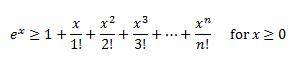# Induction proof

ptolema

## Homework Statement

prove thatusing induction

hint: use induction on n, and compare derivatives

## Homework Equations

P(n) says "1+x+x2/2!+...+xn/n!"
P(1)=1+x1/1!=1+x so P(1) is true

## The Attempt at a Solution

induction is probably my worst type of proof, i can't wrap my head around how to do it. apparently there is some some taylor series that goes with this, but we haven't done those yet. can someone please help?

## Answers and Replies

icystrike
Start from p(1) .

What is the value on left hand side?

What is the value on right hand side if you sum the first 4 terms?

After proving it is true.. assume p(n) is true such that n is an element of non-negative integers.

hence proving p(n+1) is true by the assumption that p(n) is true.

Hint: $$(n+1)^{k}\ge n^{k}$$ for k:{1,2,3,4,...,n}